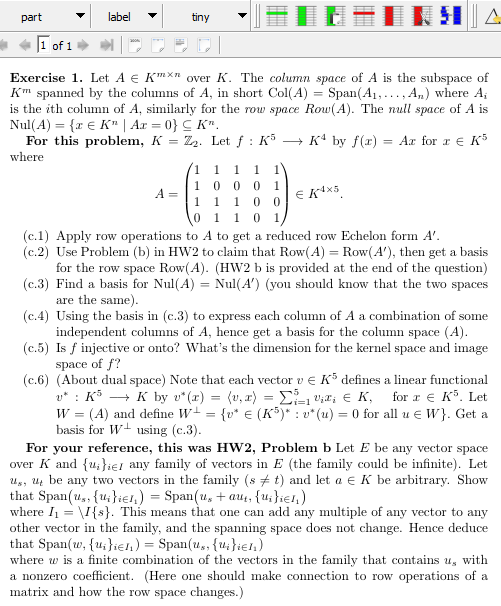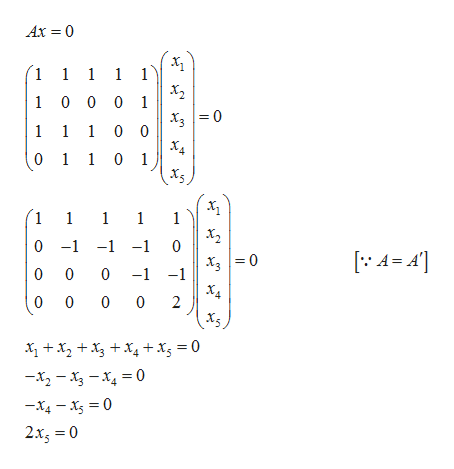labelparttiny1 of 1Exercise 1. Let A E Kn over K. The column space of A is the subspace ofKm spanned by the columns of A, in short Col(Ais the ith column of A, similarly for the rouw space Row(A). The null space of A isNul(A) E K" | Ar = 0}C K"For this problem, K = Z2. Let f : K5A) where ASpan(AK4 by f()Ar for E Kwhere111111A=10001EKXS1100 1101(c.1 Apply row operations to A to get a reduced row Echelon form A(c.2) Use Problem (b) in HW2 to claim that Row(A Row(A'), then get a basisfor the row space Row(A) HW2 b is provided at the end of the question(c.3) Find a basis for Nul(A) Nul (A') (you should know that the two spacesare the same)(c.4 Using the basis in (c.3) to express each column of A a combination of someindependent columns of A, hence get a basis for the column space (A)(c.5) Is finjective or onto? What's the dimension for the kernel space and imagespace of f?(c.6) (About dual space) Note that each vector vE K5 defines a linear functionalKs K by v*(r (v, a)- ΣΣ 0π, ε κ .(A) and define W= {v* € (K5)* : v* (u) = 0 for all u E W}. Get afor K Letbasis for W using (c.3)For your reference, this was HW2, Problem b Let E be any vector spaceover K and u;}ieI any family of vectors in E (the family could be infinite). Letus, be any two vectors in the family (st) and let a E K be arbitrary. Showthat Span(u, u}iel,) = Span(u, + aut, {u;}ie!,)where I\I{s}. This means that one can add any multiple of any vector to anyother vector in the family, and the spanning space does not change. Hence deducethat Span(w, fu}ieh) = Span(u, {u}ien)where w is a finite combination of the vectors in the family that contains u, witha nonzero coefficient. (Here one should make connection to row operations of amatrix and how the row space changes.)

Question

Please, I need a detailed and easy to understand solution to (c.4)help_outlineImage Transcriptioncloselabel part tiny 1 of 1 Exercise 1. Let A E Kn over K. The column space of A is the subspace of Km spanned by the columns of A, in short Col(A is the ith column of A, similarly for the rouw space Row(A). The null space of A is Nul(A) E K" | Ar = 0}C K" For this problem, K = Z2. Let f : K5 A) where A Span(A K4 by f() Ar for E K where 1 1 1 1 1 1 A= 1 0 0 0 1 EKXS 1 1 0 0 1 1 0 1 (c.1 Apply row operations to A to get a reduced row Echelon form A (c.2) Use Problem (b) in HW2 to claim that Row(A Row(A'), then get a basis for the row space Row(A) HW2 b is provided at the end of the question (c.3) Find a basis for Nul(A) Nul (A') (you should know that the two spaces are the same) (c.4 Using the basis in (c.3) to express each column of A a combination of some independent columns of A, hence get a basis for the column space (A) (c.5) Is finjective or onto? What's the dimension for the kernel space and image space of f? (c.6) (About dual space) Note that each vector vE K5 defines a linear functional Ks K by v*(r (v, a) - ΣΣ 0π, ε κ . (A) and define W= {v* € (K5)* : v* (u) = 0 for all u E W}. Get a for K Let basis for W using (c.3) For your reference, this was HW2, Problem b Let E be any vector space over K and u;}ieI any family of vectors in E (the family could be infinite). Let us, be any two vectors in the family (st) and let a E K be arbitrary. Show that Span(u, u}iel,) = Span(u, + aut, {u;}ie!,) where I\I{s}. This means that one can add any multiple of any vector to any other vector in the family, and the spanning space does not change. Hence deduce that Span(w, fu}ieh) = Span(u, {u}ien) where w is a finite combination of the vectors in the family that contains u, with a nonzero coefficient. (Here one should make connection to row operations of a matrix and how the row space changes.) fullscreen
Step 1

Obtain the basis o...help_outlineImage TranscriptioncloseAx 0 1 1 1 1 1 1 0 0 0 1 1 1 10 0 0 1 1 0 1 1 1 1 1 1 х, 0 -1 -1-1 0 0 0 -1 -1 ХА 0 0 0 2 x2 x4+ xg = 0 -x2-x-x 0 -x4-x 0 2xg 0 fullscreen

Want to see the full answer?

See Solution

Want to see this answer and more?

Our solutions are written by experts, many with advanced degrees, and available 24/7

See Solution
Tagged in

Other